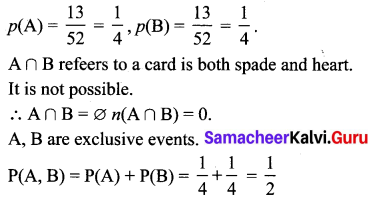Samacheer Kalvi 10th Maths Solutions Chapter 8 Statistics and Probability Additional Questions

Tamilnadu Samacheer Kalvi 10th Maths Solutions Chapter 8 Statistics and Probability Additional Questions

Question 1.
Find the standard deviation of 30, 80, 60, 70, 20, 40, 50 using the direct method.
Solution:
Direct method: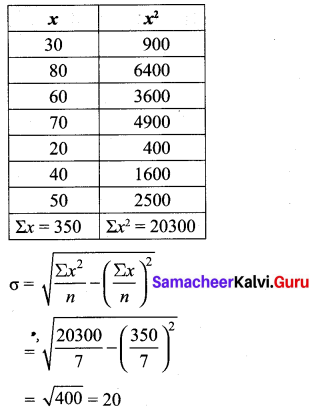Question 2.
Find the standard deviation for the following data. 5, 10, 15, 20, 25. And also find the new
S.D. if three is added to each value.
Solution: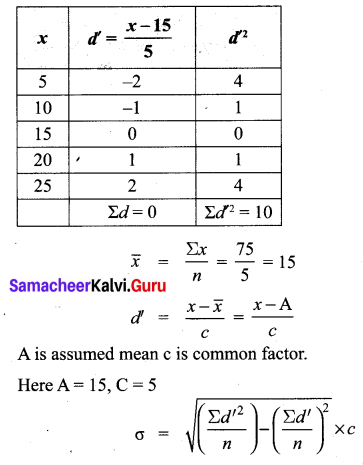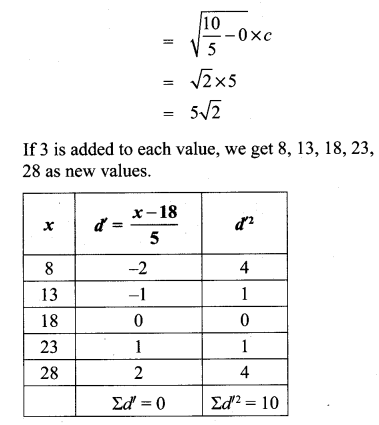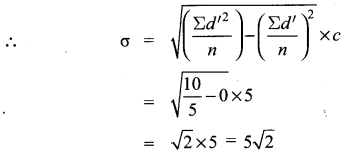S.D. doesn’t change when a number is added or subtracted to the values.Question 3.
The marks scored by 5 students in a test for 50 marks are 20, 25, 30, 35, 40. Find the S.D for the marks. If the marks are converted for 100 marks, find the S.D. for newly obtained marks.
Solution:
Let assumed mean A = 30
C = 5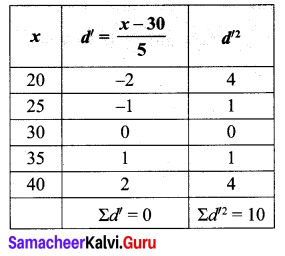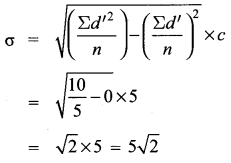To convert the values for 100, all the values will be multiplied by 2. Therefore the new values are 40, 50, 60, 70, 80.
Let A = 60,
C = 10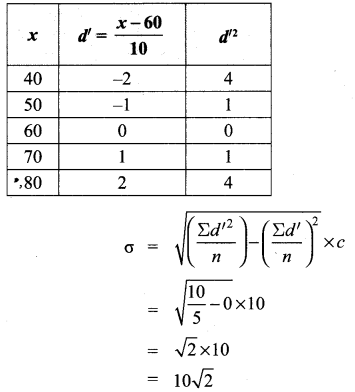S.D. also be multiplied by 2. It is also true for the division also.Question 4.Solution: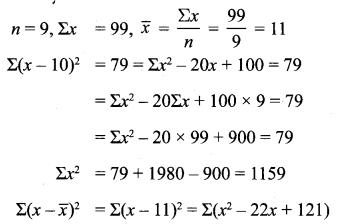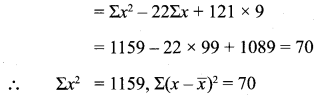Question 5.
Find the co-efficient of variation for the following data: 16, 13, 17, 21, 18.
Solution: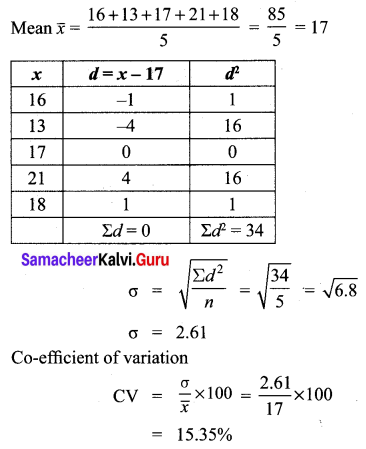Question 6.
C.V. of a data is 69%, S.D. is 15.6, then find its mean.
Solution: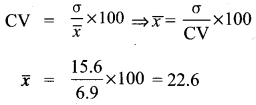Question 7.
S.D. of a data is 2102, mean is 36.6, then find its C.V.
Solution:Question 8.Which team is more consistent?
Solution: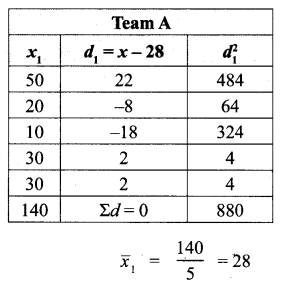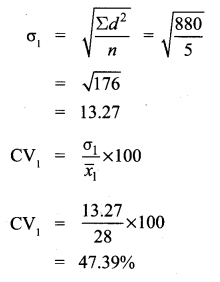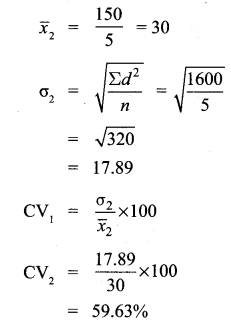∴ Team A is more consistent.Question 9.
Final the probability of choosing a spade or a heart card from a deck of cards.
Solution:
Total number of cards = 52
Event of selecting a spade card = A
Event of selecting a heart card = B
n(A) = 13,
n(B) = 13